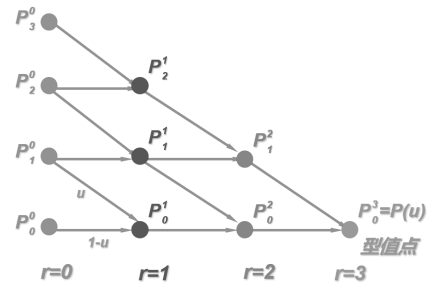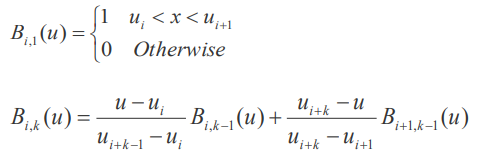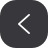# 二、算法重述

## 2.1 DDA 算法

DDA 算法，即数值差分分析算法，直接利用直线 x 或 y 方向增量 △x 或 △y，在直线投影较长的坐标轴上，以单位增量对线段离散取样，确定另一个坐标轴上最靠近线段路径的对应整数值。实际实现时，采用增量法确定这个整数值，另一个坐标轴上的增量应满足的要求是，符号使起始点具备向结束点移动的趋势，模长等于当前坐标轴投影和较长坐标轴投影的比值。

## 2.2 Bresenham 算法

Bresenham 算法利用了光栅扫描时，线段离散取样位置的有限性，只有两个可能的位置符合采样要求，于是设计整型参量来表示两个侯选位置和理想位置的偏移量，通过检测这个整型参量的符号，在侯选位置里二选一。

void drawLineByBresenham(int x1, int y1, int x2, int y2, QImage* _img, const QRgb _color) {

int stepx(1), stepy(1);
if (x1 > x2) stepx = -1;
if (y1 > y2) stepy = -1;
if (x1 == x2) { // 针对竖直线的优化
…
}
else if (y1 == y2) { // 针对水平线的优化
…
}

int x(x1), y(y1), dx(abs(x2 - x1)), dy(abs(y2 - y1));

if (dx == dy) { // 针对对角线的优化
while (x != x2) {
setPix(QPoint(x, y), _color);
x += stepx; y += stepy;
}
} // 正式开始Bresenham算法
else if (dx > dy) {
int p(2 * dy - dx), twody(2 * dy), twody_2dx(2 * (dy - dx)), i(dx);
while ((i--) >= 0) {
setPix(QPoint(x, y), _color);
x += stepx;
if (p < 0)
p += twody;
else {
p += twody_2dx; y += stepy;
}
}
}
else { // 所有变量反演
…
}
}


## 2.3 中点圆和中点椭圆算法

### 中点圆算法

fcircle(x, y) = x^2 + y^2 – r^2

• 若 fcircle(x, y) ＜ 0，(x, y)位于圆边界内；
• 若 fcircle(x, y) ＝ 0，(x, y)位于圆边界内；
• 若 fcircle(x, y) ＞ 0，(x, y)位于圆边界外。

pk = fcircle(xk+1, (yk+1 + yk) / 2) 其中 yk+1= yk-1 所以 pk = fcircle(xk+1, yk – 1/2)

pk < 0，中点在圆周边界内，选择像素位置(xk+1, yk)；

pk > 0，中点位于圆周边界外，选择像素位置(xk+1, yk-1);

pk 符号决定两候选像素中点位置(yk+2 + yk+1) / 2 的取值，

if (rx == ry) { // 标准圆算法

int x(0), y(rx), p(3 - 2 * rx); // 控制增量
while (x <= y) {
setPix(QPoint(x0 + x, y0 + y), _color);
setPix(QPoint(x0 - x, y0 - y), _color);
setPix(QPoint(x0 + x, y0 - y), _color);
setPix(QPoint(x0 - x, y0 + y), _color);
setPix(QPoint(x0 + y, y0 + x), _color);
setPix(QPoint(x0 - y, y0 + x), _color);
setPix(QPoint(x0 - y, y0 - x), _color);
setPix(QPoint(x0 + y, y0 - x), _color);
if (p >= 0) {
p += 4 * (x - y) + 10; y--;
}
else
p += 4 * x + 6;
x++;
}
}


### 中点椭圆算法

if (rx > ry) { // 中点椭圆算法

int x(0), y(ry);
double pk(0);
int ry2(ry * ry), rx2(rx * rx), rx2ry2(rx2 * ry2);

setPix(QPoint(x0 + x, y0 + y), _color);
setPix(QPoint(x0 - x, y0 - y), _color);
setPix(QPoint(x0 + x, y0 - y), _color);
setPix(QPoint(x0 - x, y0 + y), _color);
pk = ry2 - rx2 * ry + rx2 / 4.0;

while (ry2 * x <= rx2 * y) {
x++;
if (pk < 0)
pk += (2 * ry2 * x + ry2);
else {
y--; pk += (2 * ry2 * x - 2 * rx2 * y + ry2);
}
setPix(QPoint(x0 + x, y0 + y), _color);
…
}

pk = ry2 * (x + 0.5) * (x + 0.5) + rx2 * (y - 1.0) * (y - 1.0) - rx2ry2;
while (y > 0) {
y--;
if (pk > 0)
pk += (-2 * rx2 * y + rx2);
else {
x++; pk += (2 * ry2 * x - 2 * rx2 * y + rx2);
}
setPix(QPoint(x0 + x, y0 + y), _color);
…
}
}
else {
swap(x0, y0); swap(rx, ry); // 先反演所有坐标
int x(0), y(ry); // 再执行 rx > ry 的中点椭圆算法
…
}


## 2.4 图元编辑算法

### 图元旋转

const double pi = 3.1415926;
double cosr(cos(r * pi / 180.0)), sinr(sin(r * pi / 180.0));

for (auto& i : ctrlbuffer) {
int x0 = i.x(), y0 = i.y();
i.setX(x + (x0 - x) * cosr - (y0 - y) * sinr);
i.setY(y + (x0 - x) * sinr + (y0 - y) * cosr);
}


### 图元缩放

for (auto& i : ctrlbuffer) {
i.setX(x + (i.x() - x) * sx);
i.setY(y + (i.y() - y) * sy);
}


### CohenSutherland 裁剪算法

short code(0b0000);
if (point.y() > y2)
code |= 0b0001;
if (point.y() < y1)
code |= 0b0010;
if (point.x() < x1)
code |= 0b0100;
if (point.x() > x2)
code |= 0b1000;


if ((code & 0b0100)) {
setX(round(xmin));
setY(round(a.y() + (p.x() - a.x()) * m));
}
else if ((code & (0b1000))) {
p.setX(round(xmax));
setY(round(a.y() + (p.x() - a.x()) * m));
}
else if ((code & (0b0001))) {
p.setY(round(ymax));
setX(round(a.x() + (p.y() - a.y()) / m));
}
else if ((code & (0b0010))) {
p.setY(round(ymin));
setX(round(a.x() + (p.y() - a.y()) / m));
}


double m = (q.y() - p.y()) / (q.x() - p.x() + 0.000000000001);vector<QPointF> p; p.assign(input.begin(), input.end());

QPointF tmp = p; // 为了避免误差累积，全程使用浮点数计算
int div = sqrt(n); if (div < 1)div = 1;// 根据控制点个数调整步长

for (double t = 0; t <= 1 + 0.000000001; t += 0.01 / div) {
p.assign(input.begin(), input.end());
for (int i = 1; i < n; i++) { // 外层循环n-1次，即做n-1次t比分
for (int j = 0; j < n - i; j++) { //每层循环计算出n-1,n-2,...,1个切分点
p[j] = (1.0 - t) * p[j] + t * p[j + 1];
}
}
drawLineByBresenham( tmp.x(), tmp.y(), p.x(), p.y(), buffer, false);
tmp = p;
}


### 三次均匀 B 样条#### 递归函数

double Proc::bspline(double* U, double u, int i, int k) {
double result;
if (k == 1) {
if (U[i] < u && u < U[i + 1]) result = 1;
else result = 0;
}
else {
//  用条件语句体现约定： 0/0=0
result = 0;
if (i + k - 1 != i)// 要求 U[i + k - 1] - U[i] ！= 0
result += (u - U[i]) / (U[i + k - 1] - U[i]) * bspline(U, u, i, k - 1);
if (i + k != i + 1)// 要求 U[i + k] - U[i + 1] ！= 0
result += (U[i + k] - u) / (U[i + k] - U[i + 1]) * bspline(U, u, i + 1, k - 1);
}
return result;
}


#### 参数的步长迭代

for (int i = 0; i < n + k + 1; i++)
U[i] = i;
……
for (double u = U[k - 1]; u < U[n + 1]; u += 0.01 / div) {
QPointF curP(0, 0);
for (int i = 0; i < n + 1; i++)
curP += input[i] * bspline(U, u, i, k);
if (fabs(curP.x()) > 0.0001 || fabs(curP.y()) > 0.0001)
tmpBuf.push_back(curP);
}


# 三、应用设计

/*向buffer填充构成直线(x1,y1)-(x2,y2)的点，clear变量控制是否清空帧缓存*/
static void drawLineByDDA(
int x1, int y1, int x2, int y2, std::vector<QPoint>& buffer, bool clear = true
);
static void drawLineByBresenham(
int x1, int y1, int x2, int y2, std::vector<QPoint>& buffer, bool clear = true
);

/*向buffer填充构成多边形{xi,yi}的点*/
static void drawPolygonByDDA(
const std::vector<int>& xs, const std::vector<int>& ys, std::vector<QPoint>& buffer
);
static void drawPolygonByBresenham(
const std::vector<int>& xs, const std::vector<int>& ys, std::vector<QPoint>& buffer
);

/*向buffer填充构成椭圆{xi,yi}的点*/
static void drawEllipse(int x0, int y0, int rx, int ry, std::vector<QPoint>& buffer);

/*向buffer填充构成贝塞尔曲线{xi,yi}的点*/
static void drawCurveByBezier(
const std::vector<int>& xs, const std::vector<int>& ys, std::vector<QPoint>& buffer
);

/*向buffer填充构成B样条曲线{xi,yi}的点*/
static void drawCurveByBSpline(
const std::vector<int>& xs, const std::vector<int>& ys, std::vector<QPoint>& buffer
);

/*修改ctrlp为包含在矩形(x1,y1)(x2,y2)中的线段端点*/
static void clipByCohenSutherland(int x1, int y1, int x2, int y2, std::vector<QPoint>& ctrlp);
static void clipByLiangBarsky(int x1, int y1, int x2, int y2, std::vector<QPoint>& ctrlp);

/*将ctrlbuffer中的点平移(x,y)，这里的ctrlbuffer是控制点，例如直线的端点，椭圆的中心等*/
static void translate(int x, int y, std::vector<QPoint>& ctrlbuffer);

/*将ctrlbuffer中的点以(x,y)为中心顺时针旋转角度r，这里的ctrlbuffer是控制点*/
static void rotate(int x, int y, int r, std::vector<QPoint>& ctrlbuffer);

/*将ctrlbuffer中的点以(x,y)为中心放缩s，这里的ctrlbuffer是控制点，例如直线的端点，椭圆的中心等*/
static void scale(int x, int y, float sx, float sy, std::vector<QPoint>& ctrlbuffer);


bool handleCmd(std::string _cmd = std::string("resetcanvas 100 100"));
bool handleScript(const char* filename = "");


GUI 的涂鸦功能的实现细节在此不再赘述，下面介绍图元编辑的实现。

02-18218
06-128486
05-061642
11-14560
12-142688
10-26430
11-071550
05-28130
11-18573
07-131万+
07-06848
08-019200
01-291477
03-26731
09-181531
11-141743

### “相关推荐”对你有帮助么？

•非常没帮助
•没帮助
•一般
•有帮助
•非常有帮助被折叠的  条评论 为什么被折叠?到【灌水乐园】发言biyezuopinvip

¥2 ¥4 ¥6 ¥10 ¥20余额支付 (余额：-- )扫码支付获取中扫码支付点击重新获取扫码支付1.余额是钱包充值的虚拟货币，按照1:1的比例进行支付金额的抵扣。
2.余额无法直接购买下载，可以购买VIP、C币套餐、付费专栏及课程。余额充值